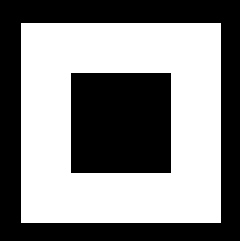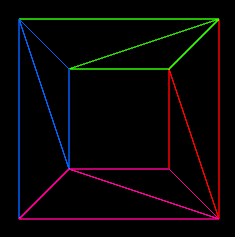# Tesselator¶

New in version 1.9.0.Warning

This is experimental and subject to change as long as this warning notice is present. Only TYPE_POLYGONS is currently supported.

Tesselator is a library for tesselating polygons, based on libtess2. It renders concave filled polygons by first tesselating them into convex polygons. It also supports holes.

## Usage¶

First, you need to create a `Tesselator` object and add contours. The first one is the external contour of your shape and all of the following ones should be holes:

```from kivy.graphics.tesselator import Tesselator

tess = Tesselator()
tess.add_contour([0, 0, 200, 0, 200, 200, 0, 200])
tess.add_contour([50, 50, 150, 50, 150, 150, 50, 150])
```

Second, call the `Tesselator.tesselate()` method to compute the points. It is possible that the tesselator won’t work. In that case, it can return False:

```if not tess.tesselate():
print("Tesselator didn't work :(")
return
```

After the tessellation, you have multiple ways to iterate over the result. The best approach is using `Tesselator.meshes` to get a format directly usable for a `Mesh`:

```for vertices, indices in tess.meshes:
vertices=vertices,
indices=indices,
mode="triangle_fan"
))
```

Or, you can get the “raw” result, with just polygons and x/y coordinates with `Tesselator.vertices()`:

```for vertices in tess.vertices:
print("got polygon", vertices)
```
class kivy.graphics.tesselator.Tesselator

Bases: `builtins.object`

Add a contour to the tesselator. It can be:

• a list of [x, y, x2, y2, …] coordinates

• a float array: array(“f”, [x, y, x2, y2, …])

• any buffer with floats in it.

element_count

Returns the number of convex polygon.

meshes

Iterate through the result of the `tesselate()` to give a result that can be easily pushed into Kivy`s Mesh object.

It’s a list of: [[vertices, indices], [vertices, indices], …]. The vertices in the format [x, y, u, v, x2, y2, u2, v2].

Careful, u/v coordinates are the same as x/y. You are responsible to change them for texture mapping if you need to.

You can create Mesh objects like that:

```tess = Tesselator()
tess.tesselate()
for vertices, indices in self.meshes:
vertices=vertices,
indices=indices,
mode="triangle_fan"))
```
tesselate(int winding_rule=WINDING_ODD, int element_type=TYPE_POLYGONS, int polysize=65535) int

Compute all the contours added with `add_contour()`, and generate polygons.

Parameters:
winding_rule: enum

The winding rule classifies a region as inside if its winding number belongs to the chosen category. Can be one of WINDING_ODD, WINDING_NONZERO, WINDING_POSITIVE, WINDING_NEGATIVE, WINDING_ABS_GEQ_TWO. Defaults to WINDING_ODD.

element_type: enum

The result type, you can generate the polygons with TYPE_POLYGONS, or the contours with TYPE_BOUNDARY_CONTOURS. Defaults to TYPE_POLYGONS.

Returns:

1 if the tessellation happened, 0 otherwise.

Return type:

int

vertex_count

Returns the number of vertex generated.

This is the raw result, however, because the Tesselator format the result for you with `meshes` or `vertices` per polygon, you’ll have more vertices in the result

vertices

Iterate through the result of the `tesselate()` in order to give only a list of [x, y, x2, y2, …] polygons.